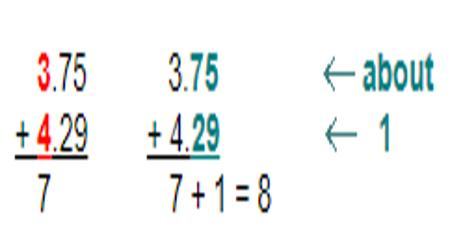Estimate the sum of decimals

To estimate means to find an answer that is close to but not exact. It is a reasonable answer to a problem.

Round each decimal term that will be added.

Example: Estimate the sum of 0.988 + 0.53
Round 0.988 + 0.53 to 1 + 0.5
Add the rounded numbers to obtain the estimated sum of 1.5
The actual sum of 0.988 + 0.53 is 1.518

Some uses of rounding are:

• Checking to see if you have enough money to buy what you want.
• Getting a rough idea of the correct answer to a problem.Example:

Charlene and Margi went to a nearby restaurant for a quick lunch. The waitress handed them the bill with the price of each lunch, but forgot to add up the total. Estimate the total bill if Charlene’s lunch was \$3.75 and Margi’s lunch was \$4.29.

Analysis: Estimation is a good tool for making a rough calculation. There are many estimation strategies that you could use to estimate the sum of these decimals. Let’s look at the front-end strategy and the rounding strategy. We will round to the nearest tenth.

Front-End Strategy

Front-end estimation is a particular way of rounding numbers to estimate sums and differences. To use front- end estimation, add or subtract only the numbers in the greatest place value. Then add the decimals rounded to the nearest tenth. Add the front digits and then adjust the estimate.3 + 4 = 7 and .75 plus .29 is about 1. Thus, \$7+ \$1 = \$8. The estimated sum is \$8.

Rounding Strategy

Round each decimal to a designated place value, then add to estimate the sum.Rounding each decimal to the nearest tenth, we get an estimated sum of 8.1 or \$8.10.

Answer: Both \$8 and \$8.10 are good estimates for the total lunch bill for Charlene and Margi.

Information Source: